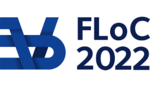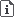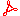FLOC 2022: FEDERATED LOGIC CONFERENCE 2022
IJCAR ON TUESDAY, AUGUST 9TH
Days:
previous day
next day
all days

View: session overviewtalk overviewside by side with other conferences

08:30-09:00Coffee & Refreshments
09:00-10:30 Session 100D: Choices, Substitutions and Formalizations
09:00

ABSTRACT. Choice logics constitute a family of propositional logics and are used for the representation of preferences, with especially qualitative choice logic (QCL) being an established formalism with numerous applications in artificial intelligence. While computational properties and applications of choice logics have been studied in the literature, only few results are known about the proof-theoretic aspects of their use. We propose a sound and complete sequent calculus for preferred model entailment in QCL, where a formula F is entailed by a QCL-theory T if F is true in all preferred models of T. The calculus is based on labeled sequent and refutation calculi, and can be easily adapted for different purposes. For instance, using the calculus as a cornerstone, calculi for other choice logics such as conjunctive choice logic (CCL) can be obtained in a straightforward way.

09:20
PRESENTER: Cezary Kaliszyk

ABSTRACT. Lash is a higher-order automated theorem prover created as a fork of the theorem prover Satallax. The basic underlying calculus of Satallax is a ground tableau calculus whose rules only use shallow information about the terms and formulas taking part in the rule. Lash uses new, efficient C representations of vital structures and operations. Most importantly, Lash uses a C representation of (normal) terms with perfect sharing along with a C implementation of normalizing substitutions. We describe the ways in which Lash differs from Satallax and the performance improvement of Lash over Satallax when used with analogous flag settings. With a 10s timeout Lash outperforms Satallax on a collection TH0 problems from the TPTP. We conclude with ideas for continuing the development of Lash.

09:35
PRESENTER: Julie Cailler

ABSTRACT. We describe Goéland, an automated theorem prover for first-order logic that relies on a concurrent search procedure to find tableau proofs, with concurrent processes corresponding to individual branches of the tableau. Since branch closure may require instantiating free variables shared across branches, processes communicate via channels to exchange information about substitutions used for closure. We present the proof-search procedure and its implementation, as well as experimental results obtained on problems from the TPTP library.

09:50

ABSTRACT. We discuss the results of our work on heuristics for generating minimal synthetic tableaux. We present this proof method for classical propositional logic and its implementation in Haskell. Based on mathematical insights and exploratory data analysis we defined a heuristics that allows to build a tableau of optimal or nearly optimal size. The proposed heuristics has been first tested on a data set with over 200 thousand of short formulas (length 12), then on a number of longer formulas (9 hundred of formulas of length 23). We describe the results of data analysis and examine some tendencies. We also confront our approach with the pigeonhole principle.

10:10
PRESENTER: Štěpán Holub

ABSTRACT. A code $X$ is not primitivity preserving if there is a primitive list $\ws \in \lists X$ whose concatenation is imprimitive. We formalize a full characterization of such codes in the binary case in the proof assistant Isabelle/HOL. Part of the formalization, interesting on its own, is a description of $\{x,y\}$-interpretations of the square $xx$ if $\abs y \leq \abs x$. We also provide a formalized parametric solution of the related equation $x^jy^k = z^\ell$

10:30-11:00Coffee Break
11:00-12:30 Session 102D: Proof Systems and Recursion
11:00

ABSTRACT. The characterizing properties of a proof-theoretical presentation of a given logic may hang on the choice of proof formalism, on the shape of the logical rules and of the sequents manipulated by a given proof system, on the underlying notion of consequence, and even on the expressiveness of its linguistic resources and on the logical framework into which it is embedded. Standard (one-dimensional) logics determined by (non-deterministic) logical matrices are known to be axiomatizable by analytic and possibly finite proof systems as soon as they turn out to satisfy a certain constraint of sufficient expressiveness. In this paper we introduce a recipe for cooking up a two-dimensional logical matrix (or B-matrix) by the combination of two (possibly partial) non-deterministic logical matrices. We will see that such a combination may result in B-matrices satisfying the property of sufficient expressiveness, even when the input matrices are not sufficiently expressive in isolate, and we will use this to show that one-dimensional logics that are not finitely axiomatizable may inhabit finitely axiomatizable two-dimensional logics, becoming, thus, finitely axiomatizable by the addition of an extra dimension. We will illustrate the said construction using a well-known logic of formal inconsistency called mCi. We will first prove that this logic is not finitely axiomatizable by a one-dimensional (generalized) Hilbert-style system. Then, taking advantage of a known 5-valued non-deterministic logical matrix for this logic, we will combine it with another one, conveniently chosen so as to give rise to a B-matrix that is axiomatized by a two-dimensional Hilbert-style system that is both finite and analytic.

11:20
Le\'sniewski's Ontology -- Proof-Theoretic CharacterizationABSTRACT. The ontology of Le\'sniewski is commonly regarded as the most comprehensive calculus of names and the theoretical basis of mereology. However, ontology was not examined by means of proof-theoretic methods so far. In the paper we prowide a characterization of elementary ontology as a sequent calculus satisfying desiderata usually formulated for decent systems in modern structural proof theory. In particular, the cut elimination theorem is proved and the version of subformula property holds for the cut-free version.

11:40
Cyclic Proofs, Hypersequents, and Transitive Closure LogicABSTRACT. We propose a cut-free cyclic system for Transitive Closure Logic (TCL) based on a form of hypersequents, suitable for automated reasoning via proof search. We show that previously proposed sequent systems are cut-free incomplete for basic validities from Kleene Algebra (KA) and Propositional Dynamic Logic (PDL), over standard translations. On the other hand, our system faithfully simulates known cyclic systems for KA and PDL, thereby inheriting their completeness results. A peculiarity of our system is its richer correctness criterion, requiring alternating automata for proof checking and necessitating a more intricate soundness argument than for traditional cyclic proofs.

12:00

ABSTRACT. I introduce substitutive sets, which are algebraic structures axiomatizing fundamental properties of variable-for-variable substitution on syntax with bindings. Substitutive sets compare favorably in some respects with the well-known foundation based on nominal sets. In particular, substitution is a more fundamental operator than the nominal swapping operator and enjoys a simpler, equationally expressed relationship with the variable-freshness predicate. Together with some natural axioms matching properties of the syntactic constructors, substitutive sets yield a truly minimalistic characterization of lambda-calculus terms as an abstract datatype, hence a recursion principle -- the first one in the literature that involves only unconditional equations on the constructors and substitution. Similarly to the case of nominal sets, an improvement of this recursion principle is possible, incorporating Barendregt’s variable convention. When interpreting syntax in semantic domains, my substitution-based recursor is easier to deploy than the nominal recursor. My results have been validated with the proof assistant Isabelle/HOL.

12:30-14:00Lunch Break

Lunches will be held in Taub lobby (CAV, CSF) and in The Grand Water Research Institute (DL, NMR, IJCAR, ITP).

14:00-15:30 Session 104D: Proof Search and Generalizations
14:00
Bayesian Ranking for Strategy Scheduling in Automated Theorem ProversPRESENTER: Chaitanya Mangla

ABSTRACT. A strategy schedule allocates time to different proof strategies in a theorem prover. We employ Bayesian statistics to propose a strategy schedule for each proof attempt. Tested on the TPTP problem library, our method yields a time saving of more than 50%. By extending this method to optimise the fixed time allocations to each strategy, we obtain a notable increase in the number of theorems proved.

14:20

ABSTRACT. Treating a saturation-based automatic theorem prover (ATP) as a Las Vegas randomized algorithm is a way to illuminate the chaotic nature of proof search and make it amenable to study by probabilistic tools. On a series of experiments with the ATP Vampire, the paper showcases some implications of this perspective for prover evaluation.

14:35

ABSTRACT. A clause $C$ is syntactically relevant in some clause set $N$, if it occurs in every refutation of $N$. A clause $C$ is syntactically semi-relevant, if it occurs in some refutation of $N$. While syntactic relevance coincides with satisfiability, i.e., if $C$ is syntactically relevant $N\setminus\{C\}$ is satisfiable, the semantic counterpart for syntactic semi-relevance was not known so far. Using the new notion of a \emph{conflict literal} we show that for independent clause sets $N$ a clause $C$ is syntactically semi-relevant in the clause set $N$ if and only if it adds to the number of conflict literals in $N$. A clause set is independent, if no clause out of the clause set is the consequence of different clauses from the clause set.

Furthermore, we relate the notion of relevance to that of a minimal unsatisfiable subset (MUS) of some independent clause set $N$. In propositional logic, a clause $C$ is relevant if it occurs in all MUSes of some clause set $N$ and semi-relevant if it occurs in some MUS. For first-order logic the characterization needs to be refined with respect to ground instances of $N$ and $C$.

14:55
Equational Unification and Matching, and Symbolic Reachability Analysis in Maude 3.2 (System Description)PRESENTER: Santiago Escobar

ABSTRACT. Equational unification and matching are fundamental mechanisms in many automated deduction applications. Supporting them efficiently for as wide as possible a class of equational theories, and in a typed manner supporting type hierarchies, benefits many applications; but this is both challenging and nontrivial. We present Maude 3.2’s efficient support of these features as well as of symbolic reachability analysis of infinite-state concurrent systems based on them.

15:10
A framework for approximate generalization in quantitative theories (ONLINE)PRESENTER: Temur Kutsia

ABSTRACT. Anti-unification aims at computing generalizations for given terms, retaining their common structure and abstracting differences by variables. We study quantitative anti-unification where the notion of the common structure is relaxed into "proximal" up to the given degree with respect to the given fuzzy proximity relation. Proximal symbols may have different names and arities. We develop a generic set of rules for computing minimal complete sets of approximate generalizations and study their properties. Depending on the characterizations of proximities between symbols and the desired forms of solutions, these rules give rise to different versions of concrete algorithms.

16:00-16:30Coffee Break
16:30-17:30 Session 108: Plenary
Chair:
16:30
SMT-based Verification of Distributed Network Control Planes

ABSTRACT. The network control plane is a complex distributed system that runs various protocols for exchanging messages between routers and selecting paths for routing traffic. Errors in control plane configurations can lead to expensive outages or critical security breaches. The last decade has seen tremendous advances in applying formal methods to ensure their correctness.

In this talk, I will describe our logic-based approach that leverages Satisfiability Modulo Theory (SMT) solvers to verify a wide variety of network correctness properties including reachability, fault-tolerance, router equivalence, and load balancing. Although this approach is general and powerful, and works well for small-sized networks (with a few hundred routers), there are scalability challenges. I will then describe some recent improvements based on key abstractions and modular assume-guarantee reasoning that have enabled our SMT-based approach to successfully handle large-sized networks (with several thousands of routers), similar to those in operation in modern data centers.

This talk describes joint work with Ryan Beckett, Ratul Mahajan, Divya Raghunathan, Timothy Alberdingk Thijm, and David Walker.